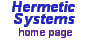User Manual forLunar Calendars and Eclipse Finder Delta TBoth the astronomical and the chronological Julian dates reflect delta T, the difference between GMT and TDT (terrestrial dynamic time, a.k.a. ephemeris time). TDT is atomic time, and thus, unlike GMT, is independent of the rotation of the Earth. Thus the times displayed for astronomical phenomena (such as full moons and eclipses) should be close to the times observed (or, for past events, would have been observed).

Unless great accuracy is required, delta T is important only for dates in the moderately distant past or future. Currently it is about one minute. It does not reach five minutes until about 1400 CE. As regards the distant future, reliable estimates of delta T cannot be made because we do not know how the Earth's rate of rotation will change.

Delta T for years past has been calculated in two ways: (i) By reference to eclipse records. (ii) By mathematical formulae. The first method gives an estimate of delta T at specific dates which is as reliable as the historical records. The second method is needed when delta T must be calculated for any given year (as in this software) but this method is not as accurate as the first.

Delta T is calculated by this program as follows: For years 1915 and later the formula of Espenak, as given on Robert van Gent's informative webpage Delta T: Approximate algorithms for historical periods is used (since this gives the best results of all formulae from the mid-20th C. to the present). [Robert van Gent's page has been temporarily withdrawn for revision, but a copy may be found here.] For years prior to 1915 delta T is calculated as the average of all non-extreme values obtained from all formulae given on that webpage except for the IAU (1952) and the Borkowski (1988) formulae (where a value is non-extreme if it does not exceed three standard deviations from the mean).

This method may not produce values for delta T as accurate as that based on historical eclipse records, but it does come close. For example, the software calculates delta T for 33 JC as as 2 hours and 48 minutes, whereas the true value (based on historical eclipse records — see the tables given in Robert van Gent's web page) is probably 2 hours and 51 minutes.